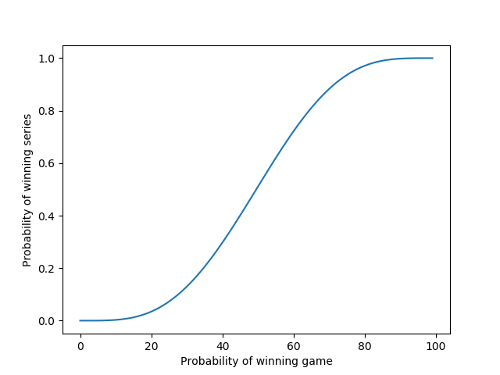# Probability of winning the World SeriesSuppose you have two baseball teams, A and B, playing in the World Series. If you like, say A stands for Houston Astros and B for Milwaukee Brewers. Suppose that in each game the probability that A wins is p, and the probability of A losing is q = 1 – p. What is the probability that A will win the series?

The World Series is a best-of-seven series, so the first team to win 4 games wins the series. Once one team wins four games there’s no point in playing the rest of the games because the series winner has been determined.

At least four games will be played, so if you win the series, you win on the 4th, 5th, 6th, or 7th game.

The probability of A winning the series after the fourth game is simply p4.

The probability of A winning after the fifth game is 4 p4 q because A must have lost one game, and it could be any one of the first four games.

The probability of A winning after the sixth game is 10 p4 q2 because A must have lost two of the first five games, and there are 10 ways to choose two items from a set of five.

Finally, the probability of A winning after the seventh game is 20 p4 q3 because A must have lost three of the first six games, and there are 20 ways to choose three items from a set of six.

The probability of winning the World Series is the sum of the probabilities of winning after 4, 5, 6, and 7 games which is

p4(1 + 4q + 10q2 + 20q3)

Here’s a plot:Obviously, the more likely you are to win each game, the more likely you are to win the series. But it’s not a straight line because the better team is more likely to win the series than to win any given game.

Now if you only wanted to compute the probability of winning the series, not the probability of winning after different numbers of games, you could pretend that all the games are played, even though some may be unnecessary to determine the winner. Then we compute the probability that a Binomial(7, p) random variable takes on a value greater than or equal to 4, which is

35p4q3 + 21p5q2 + 7p6q + p7

While looks very different than the expression we worked out above, they’re actually the same. If you stick in (1 – p) for q and work everything out, you’ll see they’re the same.

## 5 thoughts on “Probability of winning the World Series”

1. Rein Halbersma

Another fun thing to compute: the derivative w.r.t. p around p = .5 is equal to 35/16. So an extra percentage point of winning a game, translates into 2.1875 extra percentage points of winning the series.

PS the last term in the first formula should read 20 q^3, not 20 q.

2. Isaac Waisberg

Ian Stewart has a very interesting analysis of Tennis as well, in Game, Set & Math.

3. Stan Alekman

Rein,
What does w.r.t stand for?

stan alekman

4. with respect to